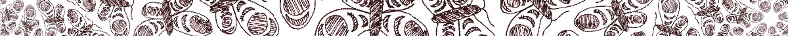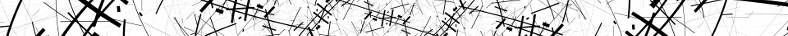# mathr / blog / #

##maths

### Fixed-point numerics revisited

A clever trick and a cleverer trick.

### Cardioid and bulb checking

Quick interior checks for Mandelbrot set escape time acceleration.

### Counting artificial neural networks

Given the total count of weights, how many shapes can the network have?

### Deep zoom theory and practice (again)

Perturbation with rebasing, and bilinear approximation, for efficient rendering of fractal images.

### Low precision high range numerics

A comparison of floating point implementations.

### Atom domains and Newton basins

A counter-example to a conjecture.

### Perturbing Nova

Affine-conjugation to move the critical point to 0 to avoid precision loss.

### Generalized series approximation

Using a computer algebra system to explore patterns.

### External ray tracing

In which various initial guesses for Newton's method are tested.

### Old Wood Dish

Analysis of a fractal artwork by JWM.

### Deep zoom theory and practice

Perturbation and rescaling for efficient rendering of fractal images.

### Optimizing zoom animations again

Finding the optimal zoom ratio when reusing the center portion.

### Spider algorithm with a path

Pull back a path from iterates of $$\theta$$ to find $$c_{\theta}$$.

### Slow mating of quadratic Julia sets

Gluing two $$z^2+c$$ quadratic Julia sets sometimes gives a $$\frac{z^2+a}{z^2+b}$$ rational Julia set.

### Fixed-point numerics

An arbitrary number of limbs before and after the point, for higher precision within a limited range.

### EVA London

Conference paper: At the Helm of the Burning Ship

### Autostereograms

An autostereogram is a single-image stereogram, designed to create the visual illusion of a three-dimensional scene from a two-dimensional image.

### Implementing a 4-pole hi/lo DJ filter

Morphing a Butterworth filter between low pass and high pass.

### Newton's method for periodic points

Rejecting lower periods by division, instead of checking later.

### Series approximation for the Burning Ship

A small region bouncing around the dynamical plane seldom touches an axis.

### Unskewing the Burning Ship

Based on the size estimate for mini-ships, use derivative matrices to calculate optimal viewing transformation.

### Enumeration of Misiurewicz points

How to determine the number of Misiurewicz points of a given (pre)period in the Mandelbrot Set.

### Non-uniform sampling

Scattering aliasing across the spectrum as white noise instead of folded frequency peaks.

### Perturbation algebra

A collection of rules for deriving perturbed iterations.

### Polar decomposition in 2D

Decomposing a 2x2 matrix into scale rotate and stretch.

### Log-polar graph paper

Fading between levels of detail to avoid huge scale differences and sharp edges.

### Möbius transformations and the Riemann sphere

Sphere rotations correspond to certain elliptic Möebius transformations, which can be interpolated in a Bézier fashion.

### Misiurewicz domain coordinates and size estimates

Do the same for Misiurewicz domains as has recently been done for atom domains.

### Atom domain coordinates

Coordinates within an atom domain surrounding a periodic nucleus in the Mandelbrot set.

### Efficent automated Julia morphing

Nucleus finding, Misiurewicz point finding, and a bit of guesswork, combine for successful O(period) automatic Julia morphing.

### A more accurate elliptic variation

Improving image quality by avoiding catastrophic cancellations.

### GULCII in Edinburgh

Untyped lambda calculus performance and seminar

### Approximating hyperbolic tangent

Computing Padé approximants isn't so hard after all.

### Approximate self-similarity

Approximate self-similarity of baby Mandelbrot sets.

### Asymptotic self-similarity

Asymptotic self-similarity about Misiurewicz points in the Mandelbrot set.

### Periodicity scan revisited

Borrowing from Mandel's "algorithm 9".

### On the precision required for size estimates

Worst-case guess improved by a factor of 8.

### Filtered atom domains

Highlighting previously invisible periodic properties in the Mandelbrot set.

### Structurally equivalent Latin squares

Canonicalization over symmetries allows them to be counted.

### Rollover 2017

Another arbitrary counter rolls over, hooray.

### Deriving the size estimate

How they did it, as far as I can tell.

### Calendar 2015 - Lung

Interlocking space filling curves.

### OEIS Diagrams

Five diagrams on the number 70.

### Finding parents in the Farey tree

An application of the extended Euclidean algorithm.

### Approximating cosine (update)

Claims of total superiority were premature.

### Fractal dimension of Julia sets

A familiar picture emerges.

### Plastic rectangles

The non-trivial partitioning of a square into 3 similar rectangles.

### PI in the Mandelbrot Set

Notes on a conjecture of Aaron Klebanoff.

### Collatz fractal

Piecewise integer recurrence translated to complex function.

### Complex squaring

Benchmarking three different implementations.

### Simpler series approximation

When thinking a bit harder beats elaborate computer code.

### Julia morphing symmetry

Rotation numbers in angled internal addresses are significant.

### Code generation for series approximation

Parallel loops for coefficient recursions.

### Automated Julia morphing

Extrapolating external angle patterns

### Floating point with extended exponent range

For when you need really big numbers without high precision.

### Möbius Infinity

Square cross-section lemniscate of Bernoulli, with a twist.

### Approximating cosine

9th order polynomial is both faster and more accurate than table lookup.

pole-zero -vs- direct form 2 -vs- pure-data

### Modes on a plate

Solving sparse eigensystems with Octave.

### Interpolating Moebius transformations

Using 2x2 matrix diagonalisation.

### Misiurewicz domains

An extension of atom domains to preperiodic points.

### Newton's method for Misiurewicz points

Rejecting lower preperiods instead of checking later.

### External angles of Misiurewicz points

Numerical investigations, hoping for a combinatorial revelation.

### A ball bouncing down steps

Physical modelling.

### Converting fractions to strings of digits

With integer, preperiodic, and periodic parts.

### Clockpunch Theorems

Do the hands of clockpunch ever form a regular hexagon?

### Perturbation glitches

Rebasing from a reference point.

### Mandelbrot Notebook

Handwritten notes on various properties.

### Stretching cusps

Conformal mapping using Möbius transformations.

### Lozenge

Interleaved dither patterns.

### Distance estimation for voting simulation visualisation

Applying a technique used for fractal rendering.

### Atom domain size estimation

A series of approximations result in a simple formula.

### Magicube

A cube of cubes that can be turned inside out.

### Islands in the hairs

Exploring external angles.

### Elpis### Haystack Situations trailer### Distance estimation for IFS fractals

Derived from continuous escape time.

### Lyapunov Space of Coupled FM Oscillators

Paper presentation at Linux Audio Conference 2013.

### Fish variations

Generalizing a tiling into hyperbolic variants.

### Rolling torus

Looping without energy loss.

### Stroking curves of constant width

An approximation for relatively narrow widths.

### Weaving a torus

out of strips of paper

### Interior coordinates in the Mandelbrot set

another representation function

### Rectangles on a triangular lattice

always contain a multiple of 4 triangles